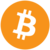btc
\$ usd

# Crypto Trading Strategy based on Elliott Wave Theory

6 mins
7 July 2020, 16:02 GMT+0000
30 June 2021, 07:19 GMT+0000
Elliott Wave Theory is a powerful tool used within technical analysis that can be used for advanced and very effective trading strategies on the cryptocurrencies market.

Ralph Nelson Elliott was an American accountant, author, and an important contributor to the study of stock markets, presenting on the table his own theory of the chart analysis called Elliott Wave Theory. Elliott’s theory basically consists of dividing the movements of the markets by their own impulses and corrections, called waves, and listing them with a sequence allowed by the relationship that one wave has with the previous and subsequent. Elliott Wave Theory is a very large but simple study that introduces us to many important chart strategies, but first, we’ll learn about Elliott’s principle.

## Elliott Wave Theory Rules

At the impulsive stage divided into 5 waves, each one of these waves is related to the others in multiple ways. The main rule tells us how waves must be distributed in size and position:
• The second wave can’t retrace more than the total length of wave 1.
• The third wave is almost always the longest, and can’t be the shortest wave of the three impulsive (in favour of trend) waves (1, 3 and 5).
• The fourth wave can’t go back further than the end of wave 1.Elliott’s wave 4 rule example The Elliott Waves can be fractalized, this means that there are smaller (micro) waves within large (macro) waves. Every wave that goes in the same trend direction is called an impulsive wave, and contains 5 microwaves; the corrective stage or corrective waves also respect that feature with 3 microwaves. We can take the same example of a bullish trend and see this better on the chart:Elliot Wave Theory macrowaves and microwaves structure for bullish example This principle is repeated on each micro or macrowave infinitely and helps us to understand that Elliott Wave Theory works on all timeframes. Let’s see an Elliott Wave Theory structure on charts for bearish example:Elliott Wave Theory principle on BTC/USD chart for bearish example

## Fibonacci Retracement and Extension tools

The Fibonacci study tells us how the market impulses and retracements are related by their length. That’s why Fibonacci can be combined with EWT and give us a powerful strategy for trading in any market. The Fibonacci retracement is a tool that shows us different levels. These levels can be achieved by the retracement or corrective stages and basically tells us where the possible end of the corrections are. To properly use this tool, we need to take a complete impulsive wave as a reference, then Fibonacci will show us the levels of retracement based on the length of this impulse.Fibonacci retracement example The most important levels for Fibonacci retracement are: 0.382, 0.50 and 0.618. They will be the key points when combining Fibonacci retracement with Elliott corrective waves. The Fibonacci extension follows the same principle of Fibonacci retracements, but in this case, the key levels will be referred to on future impulses in favour of the trend, not in corrective movements. Finally, we must move the Fibonacci levels to the initial point of the future impulse we want to study.Fibonacci extension example The most important levels for Fibonacci extension are: 0.50, 0.618, 1.00 and 1.618. They will be the key points for combining Fibonacci extension with future Elliott impulsive waves.

## How Fibonacci is related with Elliott Wave Theory

Let’s remember:
• Elliott Wave Theory consists of dividing the price movement waves, listed by numbers in the impulsive stage and by letters in the corrective stage. The impulsive stage consists of 5 waves: 1,3 and 5 for impulsive microwaves and 2, 4 for corrective microwaves.
• Fibonacci retracement tells us where the end of the corrective wave could be.
• Fibonacci extension tells us how long an impulsive wave could be.
The Elliott Wave and Fibonacci relationship is based on specific waves that have important Fibonacci extension or retracement key levels:
• During the second wave, the most important Fibonacci key levels are 0.50 and 0.618.
• During the third wave, the most important Fibonacci extension key levels are 1.00 and 1.618.
• During the fourth wave, the most important Fibonacci retracement key levels are 0.50 and 0.382.
• During the fifth wave, the most important Fibonacci extension key levels are 0.618, 1.00 and 1.618.

## The trading strategy with Elliott Wave Theory and Fibonacci study

1. Want to know more? Join our Telegram Group and get trading signals, a free trading course and daily communication with crypto fans!
2. Sign up to our newsletter for daily technical analysis, educational content and the top news stories.